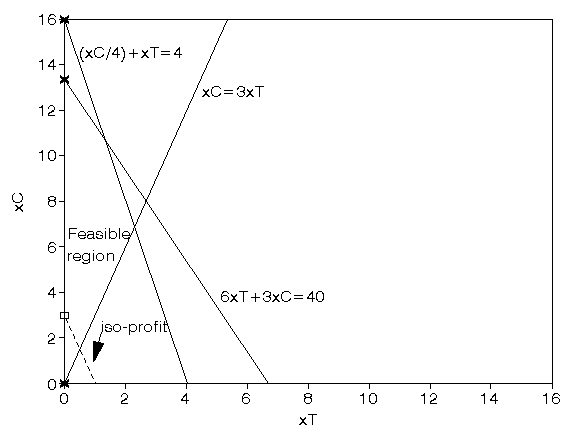# OR-Notes

## J E Beasley

OR-Notes are a series of introductory notes on topics that fall under the broad heading of the field of operations research (OR). They were originally used by me in an introductory OR course I give at Imperial College. They are now available for use by any students and teachers interested in OR subject to the following conditions.

A full list of the topics available in OR-Notes can be found here.

#### Linear programming example 1997 UG exam

A company makes two products (X and Y) using two machines (A and B). Each unit of X that is produced requires 50 minutes processing time on machine A and 30 minutes processing time on machine B. Each unit of Y that is produced requires 24 minutes processing time on machine A and 33 minutes processing time on machine B.

At the start of the current week there are 30 units of X and 90 units of Y in stock. Available processing time on machine A is forecast to be 40 hours and on machine B is forecast to be 35 hours.

The demand for X in the current week is forecast to be 75 units and for Y is forecast to be 95 units. Company policy is to maximise the combined sum of the units of X and the units of Y in stock at the end of the week.

• Formulate the problem of deciding how much of each product to make in the current week as a linear program.
• Solve this linear program graphically.

#### Solution

Let

• x be the number of units of X produced in the current week
• y be the number of units of Y produced in the current week

then the constraints are:

• 50x + 24y <= 40(60) machine A time
• 30x + 33y <= 35(60) machine B time
• x >= 75 - 30
• i.e. x >= 45 so production of X >= demand (75) - initial stock (30), which ensures we meet demand
• y >= 95 - 90
• i.e. y >= 5 so production of Y >= demand (95) - initial stock (90), which ensures we meet demand
• The objective is: maximise (x+30-75) + (y+90-95) = (x+y-50)
i.e. to maximise the number of units left in stock at the end of the week

It is plain from the diagram below that the maximum occurs at the intersection of x=45 and 50x + 24y = 2400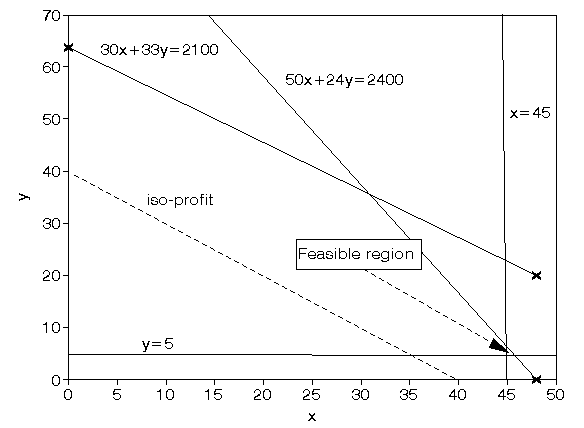Solving simultaneously, rather than by reading values off the graph, we have that x=45 and y=6.25 with the value of the objective function being 1.25

#### Linear programming example 1995 UG exam

The demand for two products in each of the last four weeks is shown below.

```                         Week
1  2  3  4
Demand - product 1    23 27 34 40
Demand - product 2    11 13 15 14
```

Apply exponential smoothing with a smoothing constant of 0.7 to generate a forecast for the demand for these products in week 5.

These products are produced using two machines, X and Y. Each unit of product 1 that is produced requires 15 minutes processing on machine X and 25 minutes processing on machine Y. Each unit of product 2 that is produced requires 7 minutes processing on machine X and 45 minutes processing on machine Y. The available time on machine X in week 5 is forecast to be 20 hours and on machine Y in week 5 is forecast to be 15 hours. Each unit of product 1 sold in week 5 gives a contribution to profit of £10 and each unit of product 2 sold in week 5 gives a contribution to profit of £4.

It may not be possible to produce enough to meet your forecast demand for these products in week 5 and each unit of unsatisfied demand for product 1 costs £3, each unit of unsatisfied demand for product 2 costs £1.

• Formulate the problem of deciding how much of each product to make in week 5 as a linear program.
• Solve this linear program graphically.

#### Solution

Note that the first part of the question is a forecasting question so it is solved below.

For product 1 applying exponential smoothing with a smoothing constant of 0.7 we get:

M1 = Y1 = 23
M2 = 0.7Y2 + 0.3M1 = 0.7(27) + 0.3(23) = 25.80
M3 = 0.7Y3 + 0.3M2 = 0.7(34) + 0.3(25.80) = 31.54
M4 = 0.7Y4 + 0.3M3 = 0.7(40) + 0.3(31.54) = 37.46

The forecast for week five is just the average for week 4 = M4 = 37.46 = 31 (as we cannot have fractional demand).

For product 2 applying exponential smoothing with a smoothing constant of 0.7 we get:

M1 = Y1 = 11
M2 = 0.7Y2 + 0.3M1 = 0.7(13) + 0.3(11) = 12.40
M3 = 0.7Y3 + 0.3M2 = 0.7(15) + 0.3(12.40) = 14.22
M4 = 0.7Y4 + 0.3M3 = 0.7(14) + 0.3(14.22) = 14.07

The forecast for week five is just the average for week 4 = M4 = 14.07 = 14 (as we cannot have fractional demand).

We can now formulate the LP for week 5 using the two demand figures (37 for product 1 and 14 for product 2) derived above.

Let

x1 be the number of units of product 1 produced

x2 be the number of units of product 2 produced

where x1, x2>=0

The constraints are:

15x1 + 7x2 <= 20(60) machine X

25x1 + 45x2 <= 15(60) machine Y

x1 <= 37 demand for product 1

x2 <= 14 demand for product 2

The objective is to maximise profit, i.e.

maximise 10x1 + 4x2 - 3(37- x1) - 1(14-x2)

i.e. maximise 13x1 + 5x2 - 125

The graph is shown below, from the graph we have that the solution occurs on the horizontal axis (x2=0) at x1=36 at which point the maximum profit is 13(36) + 5(0) - 125 = £343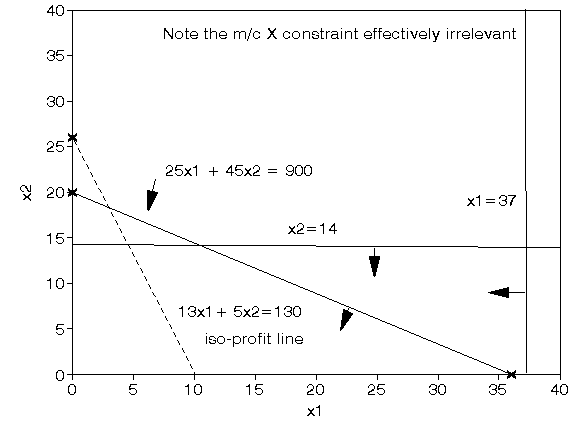#### Linear programming example 1994 UG exam

A company is involved in the production of two items (X and Y). The resources need to produce X and Y are twofold, namely machine time for automatic processing and craftsman time for hand finishing. The table below gives the number of minutes required for each item:

```         Machine time Craftsman time
Item X   13           20
Y   19           29```

The company has 40 hours of machine time available in the next working week but only 35 hours of craftsman time. Machine time is costed at £10 per hour worked and craftsman time is costed at £2 per hour worked. Both machine and craftsman idle times incur no costs. The revenue received for each item produced (all production is sold) is £20 for X and £30 for Y. The company has a specific contract to produce 10 items of X per week for a particular customer.

• Formulate the problem of deciding how much to produce per week as a linear program.
• Solve this linear program graphically.

#### Solution

Let

• x be the number of items of X
• y be the number of items of Y

then the LP is:

maximise

• 20x + 30y - 10(machine time worked) - 2(craftsman time worked)

subject to:

• 13x + 19y <= 40(60) machine time
• 20x + 29y <= 35(60) craftsman time
• x >= 10 contract
• x,y >= 0

so that the objective function becomes

maximise

• 20x + 30y - 10(13x + 19y)/60 - 2(20x + 29y)/60

i.e. maximise

• 17.1667x + 25.8667y

subject to:

• 13x + 19y <= 2400
• 20x + 29y <= 2100
• x >= 10
• x,y >= 0

It is plain from the diagram below that the maximum occurs at the intersection of x=10 and 20x + 29y <= 2100

Solving simultaneously, rather than by reading values off the graph, we have that x=10 and y=65.52 with the value of the objective function being £1866.5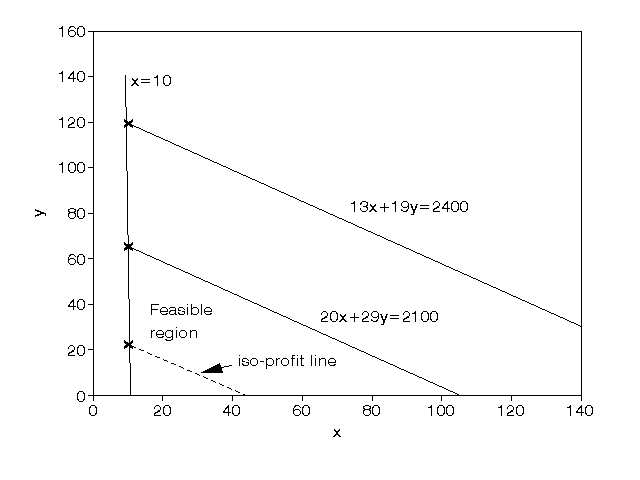#### Linear programming example 1992 UG exam

A company manufactures two products (A and B) and the profit per unit sold is £3 and £5 respectively. Each product has to be assembled on a particular machine, each unit of product A taking 12 minutes of assembly time and each unit of product B 25 minutes of assembly time. The company estimates that the machine used for assembly has an effective working week of only 30 hours (due to maintenance/breakdown).

Technological constraints mean that for every five units of product A produced at least two units of product B must be produced.

• Formulate the problem of how much of each product to produce as a linear program.
• Solve this linear program graphically.
• The company has been offered the chance to hire an extra machine, thereby doubling the effective assembly time available. What is the maximum amount you would be prepared to pay (per week) for the hire of this machine and why?

#### Solution

Let

xA = number of units of A produced

xB = number of units of B produced

then the constraints are:

12xA + 25xB <= 30(60) (assembly time)

xB >= 2(xA/5)

i.e. xB - 0.4xA >= 0

i.e. 5xB >= 2xA (technological)

where xA, xB >= 0

and the objective is

maximise 3xA + 5xB

It is plain from the diagram below that the maximum occurs at the intersection of 12xA + 25xB = 1800 and xB - 0.4xA = 0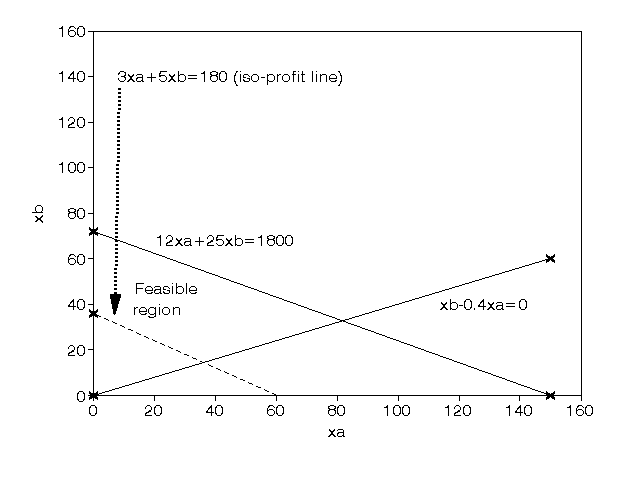Solving simultaneously, rather than by reading values off the graph, we have that:

xA= (1800/22) = 81.8

xB= 0.4xA = 32.7

with the value of the objective function being £408.9

Doubling the assembly time available means that the assembly time constraint (currently 12xA + 25xB <= 1800) becomes 12xA + 25xB <= 2(1800) This new constraint will be parallel to the existing assembly time constraint so that the new optimal solution will lie at the intersection of 12xA + 25xB = 3600 and xB - 0.4xA = 0

i.e. at xA = (3600/22) = 163.6

xB= 0.4xA = 65.4

with the value of the objective function being £817.8

Hence we have made an additional profit of £(817.8-408.9) = £408.9 and this is the maximum amount we would be prepared to pay for the hire of the machine for doubling the assembly time.

#### Linear programming example 1988 UG exam

Solve

minimise

4a + 5b + 6c

subject to

a + b >= 11

a - b <= 5

c - a - b = 0

7a >= 35 - 12b

a >= 0 b >= 0 c >= 0

#### Solution

To solve this LP we use the equation c-a-b=0 to put c=a+b (>= 0 as a >= 0 and b >= 0) and so the LP is reduced to

minimise

4a + 5b + 6(a + b) = 10a + 11b

subject to

a + b >= 11

a - b <= 5

7a + 12b >= 35

a >= 0 b >= 0

From the diagram below the minimum occurs at the intersection of a - b = 5 and a + b = 11

i.e. a = 8 and b = 3 with c (= a + b) = 11 and the value of the objective function 10a + 11b = 80 + 33 = 113.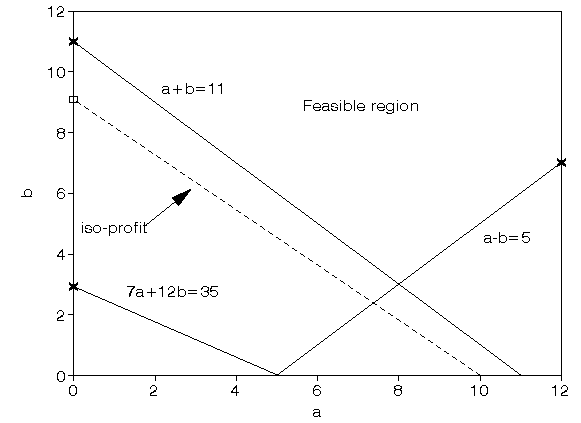#### Linear programming example 1987 UG exam

Solve the following linear program:

maximise 5x1 + 6x2

subject to

x1 + x2 <= 10

x1 - x2 >= 3

5x1 + 4x2 <= 35

x1 >= 0

x2 >= 0

#### Solution

It is plain from the diagram below that the maximum occurs at the intersection of

5x1 + 4x2 = 35 and

x1 - x2 = 3

Solving simultaneously, rather than by reading values off the graph, we have that

5(3 + x2) + 4x2 = 35

i.e. 15 + 9x2 = 35

i.e. x2 = (20/9) = 2.222 and

x1 = 3 + x2 = (47/9) = 5.222

The maximum value is 5(47/9) + 6(20/9) = (355/9) = 39.444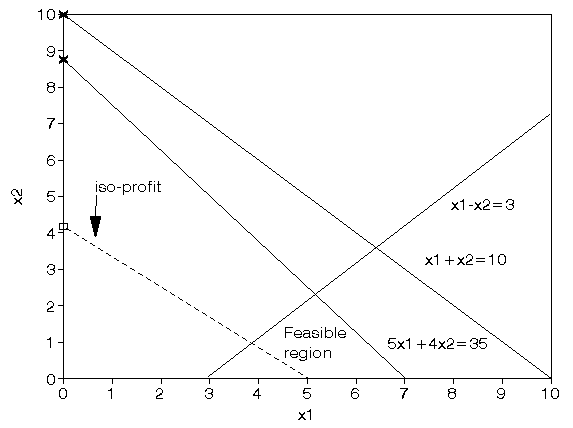#### Linear programming example 1986 UG exam

A carpenter makes tables and chairs. Each table can be sold for a profit of £30 and each chair for a profit of £10. The carpenter can afford to spend up to 40 hours per week working and takes six hours to make a table and three hours to make a chair. Customer demand requires that he makes at least three times as many chairs as tables. Tables take up four times as much storage space as chairs and there is room for at most four tables each week.

Formulate this problem as a linear programming problem and solve it graphically.

#### Variables

Let

xT = number of tables made per week

xC = number of chairs made per week

#### Constraints

• total work time

6xT + 3xC <= 40

• customer demand

xC >= 3xT

• storage space

(xC/4) + xT <= 4

• all variables >= 0

#### Objective

maximise 30xT + 10xC

The graphical representation of the problem is given below and from that we have that the solution lies at the intersection of

(xC/4) + xT = 4 and 6xT + 3xC = 40

Solving these two equations simultaneously we get xC = 10.667, xT = 1.333 and the corresponding profit = £146.667